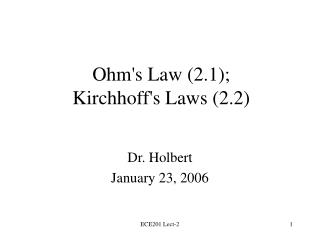Download PresentationOhm's Law (2.1); Kirchhoff's Laws (2.2)Ohm's Law (2.1); Kirchhoff's Laws (2.2) - PowerPoint PPT Presentation

Download PresentationOhm's Law (2.1); Kirchhoff's Laws (2.2)
An Image/Link below is provided (as is) to download presentation

Download Policy: Content on the Website is provided to you AS IS for your information and personal use and may not be sold / licensed / shared on other websites without getting consent from its author. While downloading, if for some reason you are not able to download a presentation, the publisher may have deleted the file from their server.

- - - - - - - - - - - - - - - - - - - - - - - - - - - E N D - - - - - - - - - - - - - - - - - - - - - - - - - - -
Presentation Transcript

1. Ohm's Law (2.1);Kirchhoff's Laws (2.2) Dr. Holbert January 23, 2006 ECE201 Lect-2

2. Resistors • A resistor is a circuit element that dissipates electrical energy (usually as heat) • Real-world devices that are modeled by resistors: incandescent light bulbs, heating elements (stoves, heaters, etc.), long wires • Resistance is measured in Ohms (W) ECE201 Lect-2

3. i(t) + The Rest of the Circuit R v(t) – Ohm’s Law • v(t) = i(t) R - or - V = I R • p(t) = i2(t) R = v2(t)/R [+ (absorbing)] ECE201 Lect-2

4. Example: a 25W Bulb • If the voltage across a 25W bulb is 120V, what is its resistance? R = V2/P = (120V)2/25W = 576  • What is the current flowing through the 25W bulb? I = V/R = 120V/576  = 0.208 A ECE201 Lect-2

5. Thought Question • When I measured the resistance of a 25W bulb, I got a value of about 40W. What’s wrong here? • Answer: The resistance of a wire increases as the temperature increases. For tungsten, the temperature coefficient of resistivity is 4.5x10-3/oK. A light bulb operates at about 5000oF. ECE201 Lect-2

6. i(t)=0 + The Rest of the Circuit v(t) – Open Circuit • What if R=? • i(t) = v(t)/R = 0 ECE201 Lect-2

7. i(t) + The Rest of the Circuit v(t)=0 – Short Circuit • What if R=0? • v(t) = R i(t) = 0 ECE201 Lect-2

8. Class Example • Learning Extension E2.1(a) • Learning Extension E2.2(a) ECE201 Lect-2

9. Kirchhoff’s Laws • Kirchhoff’s Current Law (KCL) • sum of all currents entering a node is zero • sum of currents entering node is equal to sum of currents leaving node • Kirchhoff’s Voltage Law (KVL) • sum of voltages around any loop in a circuit is zero ECE201 Lect-2

10. KCL (Kirchhoff’s Current Law) i1(t) i5(t) The sum of currents entering the node is zero: Analogy: mass flow at pipe junction i2(t) i4(t) i3(t) ECE201 Lect-2

11. Class Examples • Learning Extension E2.3(b) • Learning Extension E2.4 • Learning Extension E2.5(a) ECE201 Lect-2

12. KVL (Kirchhoff’s Voltage Law) + – • The sum of voltages around a loop is zero: • Analogy: pressure drop thru pipe loop + v2(t) + – v1(t) v3(t) – ECE201 Lect-2

13. KVL Polarity • A loop is any closed path through a circuit in which no node is encountered more than once • Voltage Polarity Convention • A voltage encountered + to - is positive • A voltage encountered - to + is negative ECE201 Lect-2

14. Class Examples • Learning Extension E2.6 • Use two different directions • Learning Extension E2.7 ECE201 Lect-2

15. Electrical Analogies (Physical) ECE201 Lect-2